Changes for Digital Image Processing and Analysis, Umbaugh, K10112, 1st Printing

Dec. 2010 and 2nd printing December 2011

CHAPTER 2:

1) P. 53, Fig 2.4-3a&c – the blue band image appears purple.

2) p. 64, 4th and 5th lines from the bottom:

It says

“…that will map the lowest value (-200)-0 and the highest value (440)-255, while…”

It should be:

“…that will map the lowest value (-200) to 0 and the highest value (440) to 255, while…”

CHAPTER 3:

1)                  p. 134, 9th line, “convexities” should be: “concavities”

CHAPTER 4:

1)      p. 145, Fig 4.2-5c, the word “strenth” should be “strength”

2) p. 158, Figure 4.2-12, the f4 mask coefficient on the lower right is a ‘0’, but should be “” , as shown here:3) p. 158, Figure 4.2-12, the f7 mask coefficient on the middle right is a ‘1’, but should be “-2”, as shown here: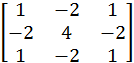4)      p. 186, second line from bottom of page:

It says “…The Harris method consists of four steps…”

It should be: ““…The Harris method consists of five steps…”

5)    p. 222, top of page SE4:

It is: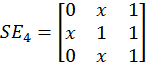It should be: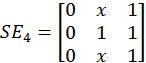6)      p. 222, bottom of page, the mask for SE8 is incorrect:

It is: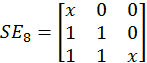It should be: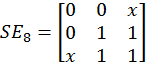7)  p. 233, the image result from Example 4.3.13 has two 0’s that should be 1’s.

It is:It should be: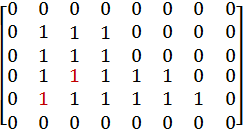8)     p. 241, add corner detectors in KEY POINTS section, after Hough.

Add the following, after Hough Transform and before Segmentation:

Corner Detection

·         Corners are points with a high rate of change in more than one direction

·         Corners are robust features

·         Corners are useful features in object tracking

·         Corners are useful in matching multiple images, for example creating 3-D models from stereo images

·         Corners are used by the human visual system to provide cues about object boundaries

Moravec corner detector: simplest, not necessarily very robust, finds points of maximum contrast (see Figure 4.2-33):Harris corner detection algorithm: 1) Blur the image with a 2-D Gaussian convolution mask, 2) Find the approximate brightness gradient in two perpendicular directions, for example use the two Prewitt or Sobel masks, 3) Blur the two brightness results with a 2-D Gaussian, 4) Find the corner response function:5) Threshold the corner response function and apply nonmaxima suppression, similar to what was done with the Canny. (see Figure 4.2-34)

Frei-Chen masks: can be used for corner detection (see Figure 4.2-35)

9) P. 250. Problem #30a) the (3,6) should be (3,5); Problem #30b) the (4,6) should be (4,5)

10)      P. 252 add this Supplementary problem:

#11) a) Apply the Moravec corner detector to the following image and show the result. b) Mark the corner points found using a threshold of 7 (do not check outer rows and columns):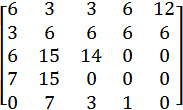Result:CHAPTER 5:

1) p. 267, Fig 5.2-2 caption, second line the equation needs more parenthesis:

It is: “…= AC|sin(πυCυC)|..”

It should be: “…= AC|[sin(πυC)]/(πυC)|...”

CHAPTER 6:

1)     p. 360,

It is:  “The most common form of the similarity measure is one that we have already seen, the vector inner product. Using our definitions for the two vectors A and B, we can define the vector inner product by the following equation:+…+) “

It should be: . The most common form of the similarity measure uses one that we have already seen, the vector inner product. Using our definitions for the two vectors A and B, we can use the normalized  vector inner product as a similarity measure, defined by the following equation: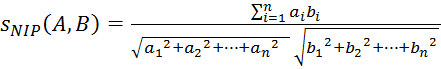2)     p. 362, Example 6.3.4, line 6 should be: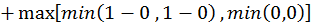(Note: line 6: “0-1” should be “1-0” and missing commas between 0,0. )

Line 8 should be: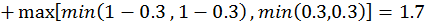(Note: line 8 , missing comma between 0.3, 0.3)

3)     p. 363, Example 6.3.5, lines 6&7 it should be: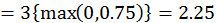(Note: change 0.75 to 1, and 0.25 to 0)

4)      p. 367, first equation, change to: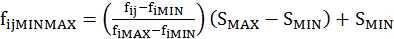Where

SMIN and SMAX are minimum and maximum value for the specified range

And

fiMIN and fiMAX are minimum and maximum for feature i

CHAPTER 8:

1)       p. 463, Line 2, it is: “is 10 + 8 + 9 + 2 + 14 + 1 + 5 + 0 = 51…”

It should be: “is 10 + 8 + 9 + 2 + 14 + 1 + 5 + 2 = 51…”

Note: the ‘0’ should be a ‘2’

2)      P. 506, 2nd line from the bottom, missing term “MIN(b,c,d)”,

It should be:

PMED(a,b,c,d,e) = (1/2)MAX[MIN(a,b,c),MIN(b,c,d),MIN(c,d,e)]

CHAPTER 9:

1) p. 559-560, the MMSE equation, please put the entire equation and variable definitions on the same page if feasible.
2) p. 569-572, it is better to have all the 9.3 figures before section 9.4.1 starts, such as was in first page proofs.

3) p. 626, exercises 21,22,24,25. In each one where it says “#19” it should be “#20”.

Chapter 10:

1)      p. 697, under “Lossless Compression Methods”, 3rd bullet, first word should be “Lossless” NOT “lossy”

2)      p. 639, Example 10.1.5, both equantions are missing a “x1024” in the denominator.

They should be: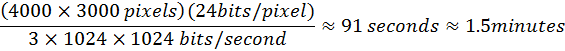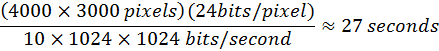CHAPTER 12:

1) p. 768&769, Fig 12.3-6 caption, middle of 5th line, should be “…FEPC_Results ..“ (underscore not dash)

APPENDIX A:

1)                  The last internet address on the page, p. 891, it is” http://www.ee.siue.edu/CVIPtools”, please change to: “http://cviptools.ece.siue.edu

APPENDIX B:

1)      p. 893, 3rd line from bottom, it is” http://www.ee.siue.edu/CVIPtools”, please change to: “http://cviptools.ece.siue.edu

APPENDIX E:

1) p. 918, “Minimum_Filter…” has many extra spaces which can be removed – there is no need for more than one space between parameters

APPENDIX F:

1) p. 923, 9th line, it is” http://www.ee.siue.edu/CVIPtools”, please change to: “http://cviptools.ece.siue.edu

INDEX

1) p. 942, the entry “CVIP-ATAT->insertion 745-729”, it should be “CVIP-ATAT->Inserting Images 745-747”

2) p. 953, please include an entry “Transforms” as follows (include page nos.):

Transforms

Cosine

Fourier

Properties

Haar

Principal components

Wavelet

3) p. 929, the entry “Basis images” needs a page number – 259

Add the following to the INDEX:

(items in parenthesis are to be listed separately, with page nos.,  under the given heading)

Additive noise, Affine transformations, Arithmetic mean filter, Bitplane run length coding, Blind deconvolution, Blocking artifact, Brightness (radiance, irradiance), Brightness adaptation, Co-occurrence matrix, Pseudocolor, Temporal resolution, Texture (classification energy map, features, Laws features, magnification of), Thiness ratio, Thresholding (average value, fuzzy c-means, hysteresis, isodata (k-means clustering), noisy images, via histogram, Otsu method), Tiepoints, Training set, Translation, Tristimulus curve, Truncated pyramid operator, Unequal length code, Utilities window,